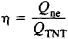# TNT Equivalent

(redirected from Tonne of TNT)
Also found in: Dictionary.
Related to Tonne of TNT: kiloton

## TNT equivalent

[¦te‚en¦tē i′kwiv·ə·lənt]
(nucleonics)
A measure of the energy released in the detonation of a nuclear weapon, expressed in terms of the weight of TNT that would release the same amount of energy when exploded; usually expressed in kilotons or megatons of TNT; based on the release of 109 calories (approximately 4.18 × 109 joules) of energy by 1 ton of TNT.

## TNT Equivalent

the mass of a conventional charge of a chemical explosive—trinitrotoluene, or TNT—whose energy of explosive decomposition is equal to the energy released in a given nuclear explosion. The TNT equivalent characterizes the explosive power of nuclear and thermonuclear charges and is calculated according to the formulawhere Qne is the energy released in the nuclear explosion and QTNT is the explosive energy of 1 ton of trinitrotoluene; it is measured in kilotons and megatons.

Site: Follow: Share:
Open / Close